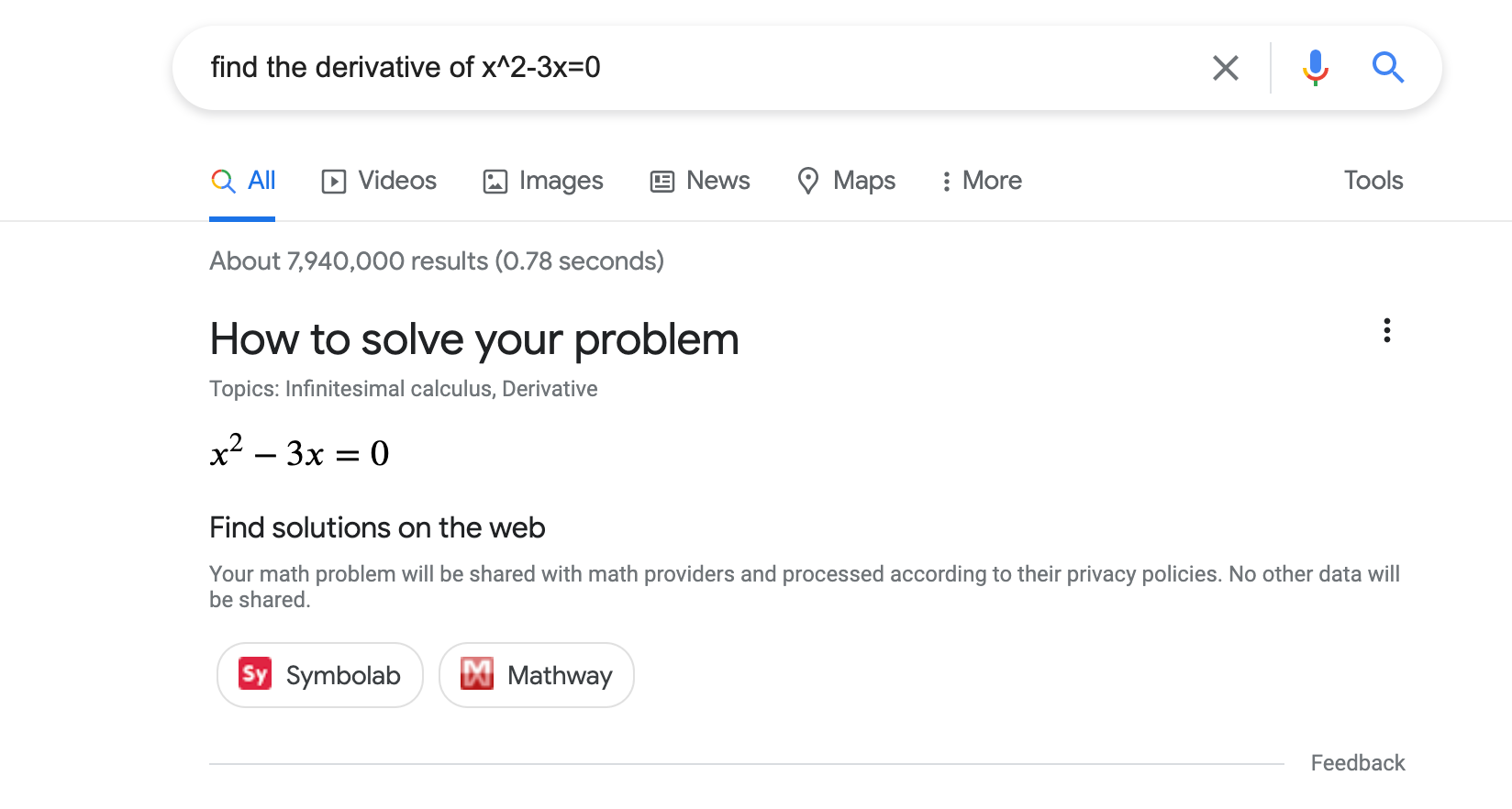# 数学求解器 (`MathSolver`) 结构化数据## 如何添加结构化数据

1. 添加必要属性。根据您使用的格式，了解在网页上的什么位置插入结构化数据
2. 遵循指南
3. 使用富媒体搜索结果测试验证您的代码，并修复所有严重错误。此外，您还可以考虑修正该工具中可能会标记的任何非严重问题，因为这些这样有助于提升结构化数据的质量（不过，要使内容能够显示为富媒体搜索结果，并非必须这么做）。
4. 部署一些包含您的结构化数据的网页，然后使用网址检查工具测试 Google 看到的网页样貌。请确保您的网页可供 Google 访问，不会因 robots.txt 文件、`noindex` 标记或登录要求而被屏蔽。如果网页看起来没有问题，您可以请求 Google 重新抓取您的网址
5. 为了让 Google 随时了解日后发生的更改，我们建议您提交站点地图Search Console Sitemap API 可以帮助您自动执行此操作。

## 示例

### 一项求解器操作

```<html>
<title>An awesome math solver</title>
<body>
<script type="application/ld+json">
[
{
"@context": "https://schema.org",
"@type": ["MathSolver", "LearningResource"],
"name": "An awesome math solver",
"url": "https://www.mathdomain.com/",
"usageInfo": "https://www.mathdomain.com/privacy",
"inLanguage": "en",
"potentialAction": [{
"@type": "SolveMathAction",
"target": "https://mathdomain.com/solve?q={math_expression_string}",
"mathExpression-input": "required name=math_expression_string",
"eduQuestionType": ["Polynomial Equation","Derivative"]
}],
"learningResourceType": "Math solver"
},
{
"@context": "https://schema.org",
"@type": ["MathSolver", "LearningResource"],
"name": "Un solucionador de matemáticas increíble",
"url": "https://es.mathdomain.com/",
"usageInfo": "https://es.mathdomain.com/privacy",
"inLanguage": "es",
"potentialAction": [{
"@type": "SolveMathAction",
"target": "https://es.mathdomain.com/solve?q={math_expression_string}",
"mathExpression-input": "required name=math_expression_string",
"eduQuestionType": ["Polynomial Equation","Derivative"]
}],
"learningResourceType": "Math solver"
}
]
</script>
</body>
</html>```

### 两项求解器操作

```<html>
<title>An awesome math solver</title>
<body>
<script type="application/ld+json">
{
"@context": "https://schema.org",
"@type": ["MathSolver", "LearningResource"],
"name": "An awesome math solver",
"url": "https://www.mathdomain.com/",
"usageInfo": "https://www.mathdomain.com/privacy",
"inLanguage": "en",
"potentialAction": [{
"@type": "SolveMathAction",
"target": "https://mathdomain.com/solve?q={math_expression_string}",
"mathExpression-input": "required name=math_expression_string",
"eduQuestionType": "Polynomial Equation"
},
{
"@type": "SolveMathAction",
"target": "https://mathdomain.com/trig?q={math_expression_string}",
"mathExpression-input": "required name=math_expression_string",
"eduQuestionType": "Trigonometric Equation"
}],
"learningResourceType": "Math solver"
}
</script>
</body>
</html>```

## 指南

### 技术指南

• `MathSolver` 结构化数据添加到您网站的首页。
• 确保您的主机负载设置能够承受频繁抓取
• 如果您在不同网址下托管了同一数学求解器的多个相同副本，请在网页的每个副本中使用规范网址

### 内容指南

• 我们不允许将宣传内容伪装成数学求解器，例如由第三方（如联属计划）发布的内容。
• 通过此功能呈现数学求解器时，您将对其准确性和质量负责。在质量审核流程中，如果我们发现一定数量的不准确数据，则根据不准确的程度，可能会从该功能中移除您的求解器，直到问题得到解决为止。适用范围：
• 求解器能够求解的数学题类型的准确性。
• 求解器声明可以求解的数学题的解法准确性。

## 结构化数据类型定义

### MathSolver

`MathSolver` 是一款工具，可以为学生、老师和其他人列出求解步骤，从而帮助他们求解数学题。请在网站的首页上使用 `MathSolver` 结构化数据。

`potentialAction`

`SolveMathAction`

```
{
"@type": "MathSolver",
"potentialAction": [{
"@type": "SolveMathAction",
"target": "https://mathdomain.com/solve?q={math_expression_string}",
"mathExpression-input": "required name=math_expression_string",
"eduQuestionType": "Polynomial Equation"
}]
}
```
`potentialAction.mathExpression-input`

`Text`

`url`

`URL`

`MathSolver` 的网址。

`usageInfo`

`URL`

```
{
"@type": "MathSolver",
"usageInfo": "https://www.mathdomain.com/privacy"
}```
`potentialAction.target`

`EntryPoint`

```
{
"@type": "MathSolver",
"potentialAction": [{
"@type": "SolveMathAction",
"target": "https://mathdomain.com/solve?q={math_expression_string}"
}]
}```

`inLanguage`

`Text`

```
{
"@type": "MathSolver",
"inLanguage": "es"
}```
`assesses`

```
{
"@type": "MathSolver",
"assesses": "Polynomial Equation"
}```
`potentialAction.eduQuestionType`

```
{
"@type": "SolveMathAction",
"eduQuestionType": "Polynomial Equation"
}
```

### LearningResource

`LearningResource` 表明标记对象是可帮助学生、老师和其他人开展教育学习的资源。请在网站的首页上使用 `LearningResource`

`learningResourceType`

`Text`

```
{
"@type": ["MathSolver", "LearningResource"],
"learningResourceType": "Math Solver"
}```

## 数学题类型定义

`MathSolver` 附有提供特定数学题讲解的 `HowTo` 时，请将以下数学题类型列表用作 `MathSolver``MathSolver.potentialAction``assesses` 字段的 `eduQuestionType`

`Absolute Value Equation`

`Algebra`

`Arc Length`

`Arithmetic`

`Biquadratic Equation`

`Calculus`

`Characteristic Polynomial`

`Circle`

`Derivative`

`Differential Equation`

`Distance`

`Eigenvalue`

`Eigenvector`

`Ellipse`

`Exponential Equation`

`Function`

`Function Composition`

`Geometry`

`Hyperbola`

`Inflection Point`

`Integral`

`Intercept`

`Limit`

`Line Equation`

`Linear Algebra`

`Linear Equation`

`Linear Inequality`

`Logarithmic Equation`

`Logarithmic Inequality`

`Matrix`

`Midpoint`

`Parabola`

`Parallel`

`Perpendicular`

`Polynomial Equation`

`Polynomial Expression`

`Polynomial Inequality`

`Quadratic Equation`

`Quadratic Expression`

`Quadratic Inequality`

`Radical Equation`

`Radical Inequality`

`Rational Equation`

`Rational Expression`

`Rational Inequality`

`Slope`

`Statistics`

`System of Equations`

`Trigonometry`

[{ "type": "thumb-down", "id": "missingTheInformationINeed", "label":"没有我需要的信息" },{ "type": "thumb-down", "id": "tooComplicatedTooManySteps", "label":"太复杂/步骤太多" },{ "type": "thumb-down", "id": "outOfDate", "label":"内容需要更新" },{ "type": "thumb-down", "id": "translationIssue", "label":"翻译问题" },{ "type": "thumb-down", "id": "samplesCodeIssue", "label":"示例/代码问题" },{ "type": "thumb-down", "id": "otherDown", "label":"其他" }]
[{ "type": "thumb-up", "id": "easyToUnderstand", "label":"易于理解" },{ "type": "thumb-up", "id": "solvedMyProblem", "label":"解决了我的问题" },{ "type": "thumb-up", "id": "otherUp", "label":"其他" }]